CBSE (Science) Class 11CBSE
Share

# HC Verma solutions for Class 11 Physics chapter 3 - Rest and Motion: Kinematics

#### HC Verma Concept of Physics Part-1 (2018-2019 Session) by H.C Verma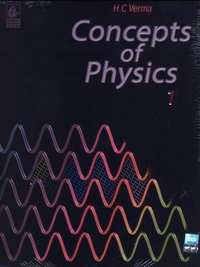## Chapter 3: Rest and Motion: Kinematics

#### HC Verma solutions for Class 11 Physics Chapter 3 Exercise Short Answers [Pages 48 - 49]

Short Answers | Q 1 | Page 48

Galileo was punished by the Church for teaching that the sun is stationary and the earth moves around it. His opponents held the view that the earth is stationary and the sun moves around it. If the absolute motion has no meaning, are the two viewpoints not equally correct or equally wrong?

Short Answers | Q 2 | Page 48

When a particle moves with constant velocity, its average velocity, its instantaneous velocity and its speed are all equal. Comment on this statement.

Short Answers | Q 3 | Page 48

A car travels at a speed of 60 km/hr due north and the other at a speed of 60 km/hr due east. Are the velocities equal? If no, which one is greater? If you find any of the questions irrelevant, explain.

Short Answers | Q 4 | Page 48

A ball is thrown vertically upward with a speed of 20 m/s. Draw a graph showing the velocity of the ball as a function of time as it goes up and then comes back.

Short Answers | Q 5 | Page 48

The velocity of a particle is towards west at an instant. Its acceleration is not towards west, not towards east, not towards north and towards south. Give an example of this type of motion .

Short Answers | Q 6 | Page 48

At which point on its path a projectile has the smallest speed?

Short Answers | Q 7 | Page 48

Two particles A and B start from rest and move for equal time on a straight line. The particle A has an acceleration a for the first half of the total time and 2a for the second half. The particle B has an acceleration 2a for the first and a for the second half. Which particle has covered larger distance?

Short Answers | Q 8 | Page 48

If a particle is accelerating, it is either speeding up or speeding down. Do you agree with this statement?

Short Answers | Q 9 | Page 48

A food packet is dropped from a plane going at an altitude of 100 m. What is the path of the packet as seen from the plane? What is the path as seen from the ground ? If someone asks "what is the actual path", what will you answer?

Short Answers | Q 10.1 | Page 48

Give example where  the velocity of a particle is zero but its acceleration is not zero.

Short Answers | Q 10.2 | Page 48

Give example where the velocity is opposite in direction to the acceleration.

Short Answers | Q 10.3 | Page 48

Give example where the velocity is perpendicular to the acceleration.

Short Answers | Q 11 | Page 48

In figure shows the x coordinate of a particle as a function of time. Find the sings of vx and ax at t = t1, t = t2 and t = t3.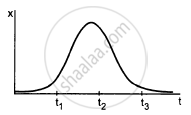Short Answers | Q 12 | Page 49

A player hits a baseball at some angle. The ball goes high up in space. The player runs and catches the ball before it hits the ground. Which of the two (the player or the ball) has greater displacement?

Short Answers | Q 13 | Page 49

The increase in the speed of a car is proportional to the additional petrol put into the engine. Is it possible to accelerate a car without putting more petrol or less petrol into the engine?

Short Answers | Q 14 | Page 49

Rain is falling vertically. A man running on the road keeps his umbrella tilted but a man standing on the street keeps his umbrella vertical to protect himself from the rain. But both of them keep their umbrella vertical to avoid the vertical sun-rays. Explain.

#### HC Verma solutions for Class 11 Physics Chapter 3 Exercise MCQ, MCQI [Pages 49 - 50]

MCQ | Q 1 | Page 49

A motor car is going due north at a speed of 50 km/h. It makes a 90° left turn without changing the speed. The change in the velocity of the car is about

• 50 km/h towards west

• 70 km/h towards south-west

•  70 km/h towards north-west

• zero

MCQ | Q 2 | Page 49

In figure shows the displacement-time graph of a particle moving on the X-axis.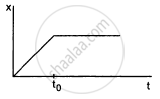• the particle is continuously going in positive x direction

•  the particle is at rest

• the velocity increases up to a time t0, and them become constant

•  the particle moves at a constant velocity up to a time t0, and then stops.

MCQ | Q 3 | Page 49

A particle has a velocity u towards east at t = 0. Its acceleration is towards west and is constant. Let xA and xB be the magnitude of displacements in the first 10 seconds and the next 10 seconds

• xA < xB

•  xA = xB

•  xA > xB

• the information is insufficient to decide the relation of xA with xB.

MCQ | Q 4 | Page 49

A person travelling on a straight line moves with a uniform velocity v1 for some time and with uniform velocity v2 for the next equal time. The average velocity v is given by

• $v = \frac{v_1 + v_2}{2}$
• $v = \sqrt{v_1 v_2}$
• $\frac{2}{v} = \frac{1}{v_1} + \frac{1}{v_2}$
• $\frac{1}{v} = \frac{1}{v_1} + \frac{1}{v_2}$
MCQ | Q 5 | Page 49

A person travelling on a straight line moves with a uniform velocity v1 for a distance x and with a uniform velocity v2 for the next equal distance. The average velocity v is given by

• $v = \frac{v_1 + v_2}{2}$

• $v = \sqrt{v_1 v_2}$

• $\frac{2}{v} = \frac{1}{v_1} + \frac{1}{v_2}$

• $\frac{1}{v} = \frac{1}{v_1} + \frac{1}{v_2}$

MCQ | Q 6 | Page 49

A stone is released from an elevator going up with an acceleration a. The acceleration of the stone after the release is

•  a upward

• (g − a) upward

•  (g − a) downward

• g downward

MCQ | Q 7 | Page 49

A person standing near the edge of the top of a building throws two balls A and B. the ball A is thrown vertically upward and B is thrown vertically downward with the same speed. The ball A hits the ground with a speed vA and the ball B this the ground with a speed vB. We have

• vA > vB

• vA < vB

• vA = vB

• the relation between vA and vB depends on height of the building above the ground.

MCQI | Q 8 | Page 49

In a projectile motion the velocity

• is always perpendicular to the acceleration

•  is never perpendicular to the acceleration

• is perpendicular to the acceleration for one instant only

•  is perpendicular to the acceleration for two instants.

MCQ | Q 9 | Page 49

Two bullets are fired simultaneously, horizontally and with different speeds from the same place. Which bullet will hit he ground first?

• the faster one

•  the slower one

•  both will reach simultaneously

• depends on the masses.

MCQ | Q 10 | Page 49

The range of a projectile fired at an angle of 15° is 50 m. If it is fired with the same speed at an angle of 45°, its range will be

• 25 m

• 37 m

• 50 m

• 100 m

MCQ | Q 11 | Page 49

Two projectiles A and B are projected with angle of projection 15° for the projectile A and 45° for the projectile B. If RA and RB be the horizontal range for the two projectiles, then

• RA < RB

• RA > RB

• RA = RB

• the information is insufficient to decide the relation of RA with RB.

MCQ | Q 12 | Page 49

A river is flowing from west to east at a speed of 5 metres per minute. A man on the south bank of the river, capable of swimming at 10 metres per minute in still water, wants to swim across the river in the shortest time. He should swim in a direction.

• due north

•  30° east of north

•  30° north of west

• 60° east of north.

MCQ | Q 13 | Page 50

In the arrangement shown in figure the ends P and Q of an inextensible string move downwards with uniform speed u. Pulleys A and B are fixed. The mass M moves upwards with a speed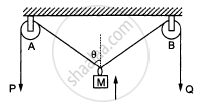•  2u cos θ

• u/cos θ

• 2u/cos θ

• u cos θ

#### HC Verma solutions for Class 11 Physics Chapter 3 Exercise MCQ [Pages 50 - 51]

MCQ | Q 1 | Page 50

Consider the motion of the tip of the minute hand of a clock. In one hour
(a) the displacement is zero
(b) the distance covered is zero
(c) the average speed is zero
(d) the average velocity is zero

MCQ | Q 2 | Page 50

A particle moves along the X-axis as x = u (t − 2 s) + a (t − 2 s)2.
(a) the initial velocity of the particle is u
(b) the acceleration of the particle is a
(c) the acceleration of the particle is 2a
(d) at t = 2 s particle is at the origin.

MCQ | Q 3 | Page 50

Pick the correct statements:
(a) Average speed of a particle in a given time is never less than the magnitude of the average velocity.
(b) It is possible to have a situation in which

$\left| \frac{d \vec{v}}{dt} \right| \neq 0 \text{ but } \frac{d}{dt} = \left| \vec{v} \right| = 0$
(c) The average velocity of a particle is zero in a time interval. It is possible that the instantaneous velocity is never zero is the interval.
(d) The average velocity of a particle moving on a straight line is zero in a time interval. It is possible that the instantaneous velocity is never zero in the interval. (Infinite accelerations are not allowed).
MCQ | Q 4 | Page 50

An object may have
(a) varying speed without having varying velocity
(b) varying velocity without having varying speed
(c) nonzero acceleration without having varying velocity
(d) nonzero acceleration without having varying speed.

MCQ | Q 5 | Page 50

Mark the correct statements for a particle going on a straight line:
(a) If the velocity and acceleration have opposite sign, the object is slowing down.
(b) If the position and velocity have opposite sign the particle is moving towards the origin.
(c) If the velocity is zero at an instant, the acceleration should also be zero at that instant.
(d) If the velocity is zero for a time interval, the acceleration is zero at any instant within the time interval.

MCQ | Q 6 | Page 50

The velocity of a particle is zero at t = 0.
(a) The acceleration at t = 0 must be zero.
(b) The acceleration at t = 0 may be zero.
(c) If the acceleration is zero from t = 0 to t = 10 s, the speed is also zero in this interval.
(d) If the speed is zero from t = 0 to t = 10 s the acceleration is also zero in this interval.

MCQ | Q 7 | Page 50

Mark the correct statements:

• The magnitude of the velocity of a particle is equal to its speed.

• The magnitude of average velocity in an interval is equal to its average speed in that interval.

• It is possible to have a situation in which the speed of a particle is always zero but the average speed is not zero.

• It is possible to have a situation in which the speed of the particle is never zero but the average speed in an interval is zero.

MCQ | Q 8 | Page 50

The velocity-time plot for a particle moving on a straight line is shown in the figure.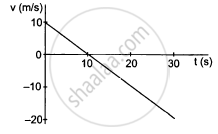(a) The particle has a constant acceleration.
(b) The particle has never turned around.
(c) The particle has zero displacement.
(d) The average speed in the interval 0 to 10 s is the same as the average speed in the interval 10 s to 20 s.

MCQ | Q 9 | Page 50

In figure shows the position of a particle moving on the X-axis as a function of time.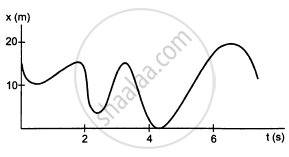• The particle has come to rest 6 times.

• The maximum speed is at t = 6s.

• The velocity remains positive for t = 0 to t = 6s.

• The average velocity for the total period shown is negative.

MCQ | Q 10 | Page 51

The accelerations of a particle as seen from two frames S1 and S2 have equal magnitude 4 m/s2.

• The frames must be at rest with respect to each other.

• The frames may be moving with respect to each other.

• The acceleration of S2 with respect to S1 may either be zero of 8 m/s2.

• The acceleration of S2 with respect to S1 may be anything between zero and 8 m/s2.

#### HC Verma solutions for Class 11 Physics Chapter 3 [Pages 51 - 54]

Q 1 | Page 51

A man has to go 50 m due north, 40 m due east and 20 m due south to reach a field. (a) What distance he has to walk to reach the field? (b) What is his displacement from his house to the field?

Q 2 | Page 51

A particle starts from the origin, goes along the X-axis to the point (20 m, 0) and then return along the same line to the point (−20 m, 0). Find the distance and displacement of the particle during the trip.

Q 3.1 | Page 51

It is 260 km from Patna to Ranchi by air and 320 km by road. An aeroplane takes 30 minutes to go from Patna to Ranchi whereas a delux bus takes 8 hours.  Find the average speed of the plane.

Q 3.2 | Page 51

It is 260 km from Patna to Ranchi by air and 320 km by road. An aeroplane takes 30 minutes to go from Patna to Ranchi whereas a delux bus takes 8 hours.   Find the average speed of the bus.

Q 3.3 | Page 51

It is 260 km from Patna to Ranchi by air and 320 km by road. An aeroplane takes 30 minutes to go from Patna to Ranchi whereas a delux bus takes 8 hours. Find the average velocity of the plane.

Q 3.4 | Page 51

It is 260 km from Patna to Ranchi by air and 320 km by road. An aeroplane takes 30 minutes to go from Patna to Ranchi whereas a delux bus takes 8 hours. Find the average velocity of the bus.

Q 4.1 | Page 51

When a person leaves his home for sightseeing by his car, the meter reads 12352 km. When he returns home after two hours the reading is 12416 km. What is the average speed of the care during this period?

Q 4.2 | Page 51

When a person leaves his home for sightseeing by his car, the meter reads 12352 km. When he returns home after two hours the reading is 12416 km. What is the average of velocity?

Q 5 | Page 51

An athlete takes 2.0 s to reach his maximum speed of 18.0 km/h. What is the magnitude of this average acceleration.

Q 6 | Page 51

The speed of a car as a function of time is shown in the following figure. Find the distance travelled by the car in 8 seconds and its acceleration.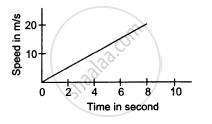Q 7 | Page 51

The acceleration of a cart started at t = 0, varies with time as shown in the following figure. Find the distance travelled in 30 seconds and draw the position-time graph.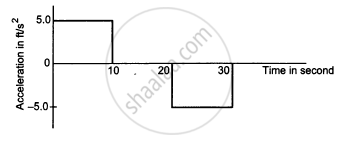Q 8.1 | Page 51

In the following figure Shows the graph of velocity versus time for a particle going along the X-axis. Find the acceleration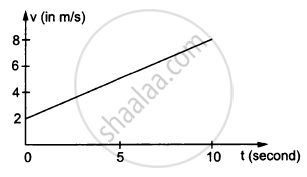Q 8.2 | Page 51

In the following figure Shows the graph of velocity versus time for a particle going along the X-axis. Find the distance travelled in 0 to 10sQ 8.3 | Page 51

In the following figure Shows the graph of velocity versus time for a particle going along the X-axis. Find the displacement in 0 to 10 s.Q 9.1 | Page 51

In the following figure shows the graph of the x-coordinate of a particle going along the X-axis as a function of time. Find the average velocity during 0 to 10 s,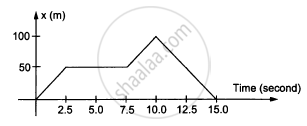Q 9.2 | Page 51

In the following figure shows the graph of the x-coordinate of a particle going along the X-axis as a function of time. Find instantaneous velocity at 2, 5, 9 and 12s.Q 10 | Page 51

From the velocity-time plot shown in the following figure, find the distance travelled by the particle during the first 40 seconds. Also find the average velocity during this period.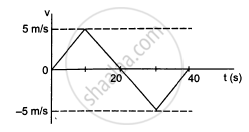Q 11 | Page 52

In the following figure  shows x-t graph of a particle. Find the time t such that the average velocity of the particle during the period 0 to t is zero.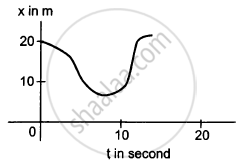Q 12 | Page 52

Figure A particle starts from a point A and travels along the solid curve shown in the following figure. Find approximately the position B of the particle such that the average velocity between the position A and B has the same direction as the instantaneous velocity at B.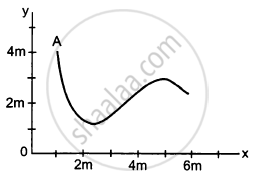Q 13 | Page 52

An object having a velocity 4.0 m/s is accelerated at the rate of 1.2 m/s2 for 5.0 s. Find the distance travelled during the period of acceleration.

Q 14 | Page 52

A person travelling at 43.2 km/h applies the brake giving a deceleration of 6.0 m/s2 to his scooter. How far will it travel before stopping?

Q 15.1 | Page 52

A train starts from rest and moves with a constant acceleration of 2.0 m/s2 for half a minute. The brakes are then applied and the train comes to rest in one minute. Find the total distance moved by the train .

Q 15.2 | Page 52

A train starts from rest and moves with a constant acceleration of 2.0 m/s2 for half a minute. The brakes are then applied and the train comes to rest in one minute. Find  the maximum speed attained by the train .

Q 15.3 | Page 52

A train starts from rest and moves with a constant acceleration of 2.0 m/s2 for half a minute. The brakes are then applied and the train comes to rest in one minute. Find the position(s) of the train at half the maximum speed.

Q 16 | Page 52

A bullet travelling with a velocity of 16 m/s penetrates a tree trunk and comes to rest in 0.4 m. Find the time taken during the retardation.

Q 17 | Page 52

A bullet going with speed 350 m/s enters a concrete wall and penetrates a distance of 5.0 cm before coming to rest. Find the deceleration.

Q 18.1 | Page 52

A particle starting from rest moves with constant acceleration. If it takes 5.0 s to reach the speed 18.0 km/h find the average velocity during this period .

Q 18.2 | Page 52

A particle starting from rest moves with constant acceleration. If it takes 5.0 s to reach the speed 18.0 km/h find the distance travelled by the particle during this period.

Q 19 | Page 52

A driver takes 0.20 s to apply the brakes after he sees a need for it. This is called the reaction time of the driver. If he is driving a car at a speed of 54 km/h and the brakes cause a deceleration of 6.0 m/s2, find the distance travelled by the car after he sees the need to put the brakes on.

Q 20 | Page 52

Complete the following table:

 Car Model Driver XReaction time 0.20 s Driver YReaction time 0.30 s A (deceleration on hard braking = 6.0 m/s2) Speed = 54 km/hBraking distancea = ............Total stopping distanceb = ............ Speed = 72 km/hBraking distancec = ...........Total stopping distanced = ............ B (deceleration on hard braking = 7.5 m/s2) Speed = 54 km/hBreaking distancee = ...........Total stopping distancef = ............ Speed 72 km/hBraking distanceg = .............Total stopping distanceh = ............

Q 21 | Page 52

A police jeep is chasing a culprit going on a motorbike. The motorbike crosses a turning at a speed of 72 km/h. The jeep follows it at a speed of 90 km/h, crossing the turning ten seconds later than the bike. Assuming that they travel at constant speeds, how far from the turning will the jeep catch up with the bike?

Q 22 | Page 52

A car travelling at 60 km/h overtakes another car travelling at 42 km/h. Assuming each car to be 5.0 m long, find the time taken during the overtake and the total road distance used for the overtake.

Q 23.1 | Page 52

A ball is projected vertically upward with a speed of 50 m/s. Find the maximum height.

Q 23.2 | Page 52

A ball is projected vertically upward with a speed of 50 m/s. Find the time to reach the maximum height .

Q 23.3 | Page 52

A ball is projected vertically upward with a speed of 50 m/s. Find the speed at half the maximum height. Take g = 10 m/s2

Q 24 | Page 52

A ball is dropped from a balloon going up at a speed of 7 m/s. If the balloon was at a height 60 m at the time of dropping the ball, how long will the ball take in reaching the ground?

Q 25.1 | Page 52

A stone is thrown vertically upward with a speed of 28 m/s. Find the maximum height reached by the stone.

Q 25.2 | Page 52

A stone is thrown vertically upward with a speed of 28 m/s.Find its velocity one second before it reaches the maximum height.

Q 25.3 | Page 52

A stone is thrown vertically upward with a speed of 28 m/s. change if the initial speed is more than 28 m/s such as 40 m/s or 80 m/s ?

Q 26 | Page 52

A person sitting on the top of a tall building is dropping balls at regular intervals of one second. Find the positions of the 3rd, 4th and 5th ball when the 6th ball is being dropped.

Q 27 | Page 52

A healthy youngman standing at a distance of 7 m from a 11.8 m high building sees a kid slipping from the top floor. With what speed (assumed uniform) should he run to catch the kid at the arms height (1.8 m)?

Q 28 | Page 52

An NCC parade is going at a uniform speed of 6 km/h through a place under a berry tree on which a bird is sitting at a height of 12.1 m. At a particular instant the bird drops a berry. Which cadet (give the distance from the tree at the instant) will receive the berry on his uniform?

Q 29 | Page 52

A ball is dropped from a height. If it takes 0.200 s to cross the last 6.00 m before hitting the ground, find the height from which it was dropped. Take g = 10 m/s2.

Q 30 | Page 52

A ball is dropped from a height of 5 m onto a sandy floor and penetrates the sand up to 10 cm before coming to rest. Find the retardation of the ball is sand assuming it to be uniform.

Q 31 | Page 52

An elevator is descending with uniform acceleration. To measure the acceleration, a person in the elevator drops a coin at the moment the elevator starts. The coin is 6 ft above the floor of the elevator at the time it is dropped. The person observes that the coin strikes the floor in 1 second. Calculate from these data the acceleration of the elevator.

Q 32.1 | Page 52

A ball is thrown horizontally from a point 100 m above the ground with a speed of 20 m/s. Find the time it takes to reach the ground .

Q 32.2 | Page 52

A ball is thrown horizontally from a point 100 m above the ground with a speed of 20 m/s. Find the horizontal distance it travels before reaching the ground .

Q 32.3 | Page 52

A ball is thrown horizontally from a point 100 m above the ground with a speed of 20 m/s. Find the velocity (direction and magnitude) with which it strikes the ground.

Q 33.1 | Page 52

A ball is thrown at a speed of 40 m/s at an angle of 60° with the horizontal. Find the maximum height reached  .

Q 33.2 | Page 52

A ball is thrown at a speed of 40 m/s at an angle of 60° with the horizontal. Find   the range of the ball. Take g = 10 m/s2

Q 34 | Page 52

In a soccer practice session the football is kept at the centre of the filed 40 yards from the 10 ft high goalposts. A goal is attempted by kicking the football at a speed of 64 ft/s at an angle of 45° to the horizontal. Will the ball reach the goal post?

Q 35 | Page 52

A popular game in Indian villages is goli which is played with small glass balls called golis. The goli of one player is situated at a distance of 2.0 m from the goli of the second player. This second player has to project his goli by keeping the thumb of the left hand at the place of his goli, holding the goli between his two middle fingers and making the throw. If the projected goli hits the goli of the first player, the second player wins. If the height from which the goli is projected is 19.6 cm from the ground and the goli is to be projected horizontally, with what speed should it be projected so that it directly hits the stationery goli without falling on the ground earlier?

Q 36 | Page 52

In the following figure shows a 11.7 ft wide ditch with the approach roads at an angle of 15° with the horizontal. With what minimum speed should a motorbike be moving on the road so that it safely crosses the ditch?

Assume that the length of the bike is 5 ft, and it leaves the road when the front part runs out of the approach road.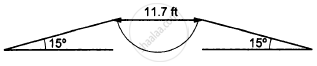Q 37 | Page 52

A person standing on the top of a cliff 171 ft high has to throw a packet to his friend standing on the ground 228 ft horizontally away. If he throws the packet directly aiming at the friend with a speed of 15.0 ft/s, how short will the packet fall?

Q 38 | Page 53

A ball is projected from a point on the floor with a speed of 15 m/s at an angle of 60° with the horizontal. Will it hit a vertical wall 5 m away from the point of projection and perpendicular to the plane of projection without hitting the floor? Will the answer differ if the wall is 22 m away?

Q 39 | Page 53

Find the average velocity of a projectile between the instants it crosses half the maximum height. It is projected with a speed u at an angle θ with the horizontal.

Q 40 | Page 53

A bomb is dropped from a plane flying horizontally with uniform speed. Show that the bomb will explode vertically below the plane. Is the statement true if the plane flies with uniform speed but not horizontally?

Q 41 | Page 53

A boy standing on a long railroad car throws a ball straight upwards. The car is moving on the horizontal road with an acceleration of 1 m/s2 and the projection velocity in the vertical direction is 9.8 m/s. How far behind the boy will the ball fall on the car?

Q 42 | Page 53

A staircase contains three steps each 10 cm high and 20 cm wide (in the following figure). What should be the minimum horizontal velocity of a ball rolling of the uppermost plane so as to hit directly the lowest plane ?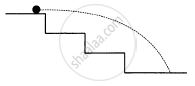Q 43.1 | Page 53

A person is standing on a truck moving with a constant velocity of 14.7 m/s on a horizontal road. The man throws a ball in such a way that it returns to the truck after the truck has moved 58.8 m. Find the speed and the angle of projection as seen from the truck .

Q 43.2 | Page 53

A person is standing on a truck moving with a constant velocity of 14.7 m/s on a horizontal road. The man throws a ball in such a way that it returns to the truck after the truck has moved 58.8 m. Find the speed and the angle of projection  as seen from the road.

Q 44 | Page 53

The benches of a gallery in a cricket stadium are 1 m wide and 1 m high. A batsman strikes the ball at a level one metre above the ground and hits a mammoth sixer. The ball starts at 35 m/s at an angle of 53° with the horizontal. The benches are perpendicular to the plane of motion and the first bench is 110 m from the batsman. On which bench will the ball hit?

Q 45 | Page 53

A man is sitting on the shore of a river. He is in the line of 1.0 m long boat and is 5.5 m away from the centre of the boat. He wishes to throw an apple into the boat. If he can throw the apple only with a speed of 10 m/s, find the minimum and maximum angles of projection for successful shot. Assume that the point of projection and the edge of the boat are in the same horizontal level.

Q 46.1 | Page 54

A river 400 m wide is flowing at a rate of 2.0 m/s. A boat is sailing at a velocity of 10 m/s with respect to the water, in a direction perpendicular to the river. Find the time taken by the boat to reach the opposite bank.

Q 46.2 | Page 54

A river 400 m wide is flowing at a rate of 2.0 m/s. A boat is sailing at a velocity of 10 m/s with respect to the water, in a direction perpendicular to the river. How far from the point directly opposite to the starting point does the boat reach the opposite bank?

Q 47.1 | Page 54

A swimmer wishes to cross a 500 m wide river flowing at 5 km/h. His speed with respect to water is 3 km/h. If he heads in a direction making an angle θ with the flow, find the time he takes to cross the river.

Q 47.2 | Page 54

A swimmer wishes to cross a 500 m wide river flowing at 5 km/h. His speed with respect to water is 3 km/h.  Find the shortest possible time to cross the river.

Q 48 | Page 54

Consider the situation of the previous problem. The man has to reach the other shore at the point directly opposite to his starting point. If he reaches the other shore somewhere else, he has to walk down to this point. Find the minimum distance that he has to walk.

Q 49.1 | Page 54

An aeroplane has to go from a point A to another point B, 500 km away due 30° east of north. A wind is blowing due north at a speed of 20 m/s. The air-speed of the plane is 150 m/s. Find the direction in which the pilot should head the plane to reach the point B.

Q 49.2 | Page 54

An aeroplane has to go from a point A to another point B, 500 km away due 30° east of north. A wind is blowing due north at a speed of 20 m/s. The air-speed of the plane is 150 m/s. Find the time taken by the plane to go from A to B.

Q 50 | Page 54

Two friends A and B are standing a distance x apart in an open field and wind is blowing from A to B. A beat a drum and B hears the sound t1 time after he sees the event. A and B interchange their positions and the experiment is repeated. This time B hears the drum timer after he sees the event. Calculate the velocity of sound in still air v and the velocity of wind u. Neglect the time light takes in travelling between the friends.

Q 51 | Page 54

Suppose A and B in the previous problem change their positions in such a way that the line joining them becomes perpendicular to the direction of wind while maintaining the separation x. What will be the time B finds between seeing and hearing the drum beating by A?

Q 52 | Page 54

Six particles situated at the corner of a regular hexagon of side a move at a constant speed v. Each particle maintains a direction towards the particle at the next corner. Calculate the time the particles will take to meet each other.

## Chapter 3: Rest and Motion: Kinematics

#### HC Verma Concept of Physics Part-1 (2018-2019 Session) by H.C Verma## HC Verma solutions for Class 11 Physics chapter 3 - Rest and Motion: Kinematics

HC Verma solutions for Class 11 Physics chapter 3 (Rest and Motion: Kinematics) include all questions with solution and detail explanation. This will clear students doubts about any question and improve application skills while preparing for board exams. The detailed, step-by-step solutions will help you understand the concepts better and clear your confusions, if any. Shaalaa.com has the CBSE Concept of Physics Part-1 (2018-2019 Session) by H.C Verma solutions in a manner that help students grasp basic concepts better and faster.

Further, we at Shaalaa.com provide such solutions so that students can prepare for written exams. HC Verma textbook solutions can be a core help for self-study and acts as a perfect self-help guidance for students.

Concepts covered in Class 11 Physics chapter 3 Rest and Motion: Kinematics are Acceleration, Relative Velocity, Kinematic Equations for Uniformly Accelerated Motion, Elementary Concepts of Differentiation and Integration for Describing Motion, Uniform and Non-Uniform Motion, Position, Path Length and Displacement, Average Velocity and Average Speed, Instantaneous Velocity and Speed, Uniformly Accelerated Motion, Position-time, Velocity-time and Acceleration-time Graphs, Motion in a Straight Line - Position-time Graph, Relations for Uniformly Accelerated Motion (Graphical Treatment), Introduction.

Using HC Verma Class 11 solutions Rest and Motion: Kinematics exercise by students are an easy way to prepare for the exams, as they involve solutions arranged chapter-wise also page wise. The questions involved in HC Verma Solutions are important questions that can be asked in the final exam. Maximum students of CBSE Class 11 prefer HC Verma Textbook Solutions to score more in exam.

Get the free view of chapter 3 Rest and Motion: Kinematics Class 11 extra questions for Physics and can use Shaalaa.com to keep it handy for your exam preparation

S